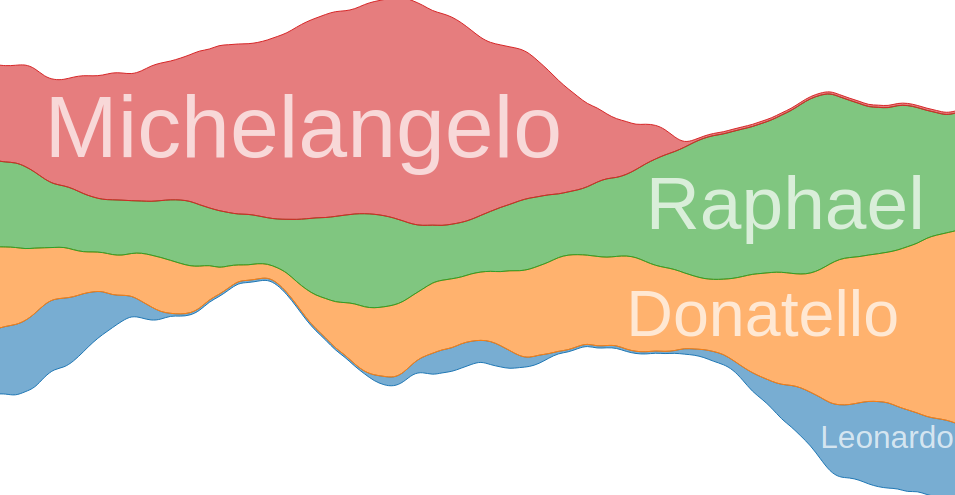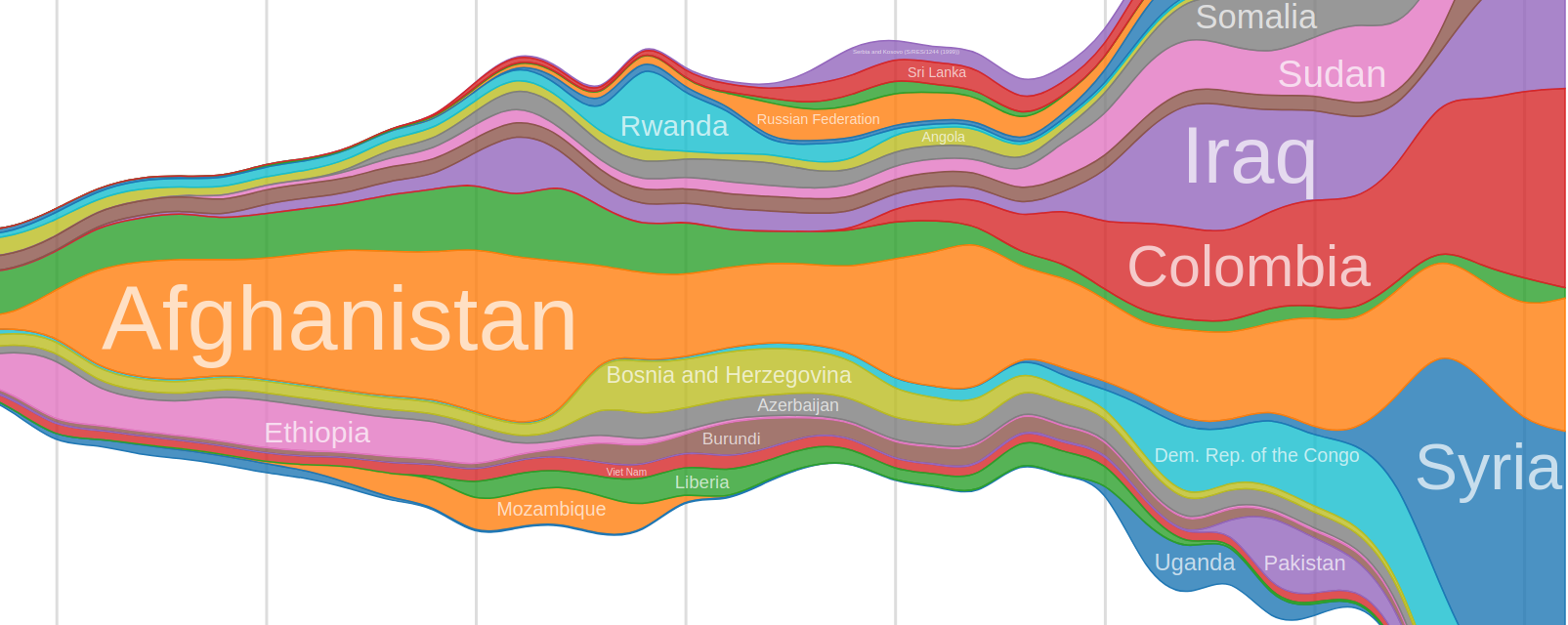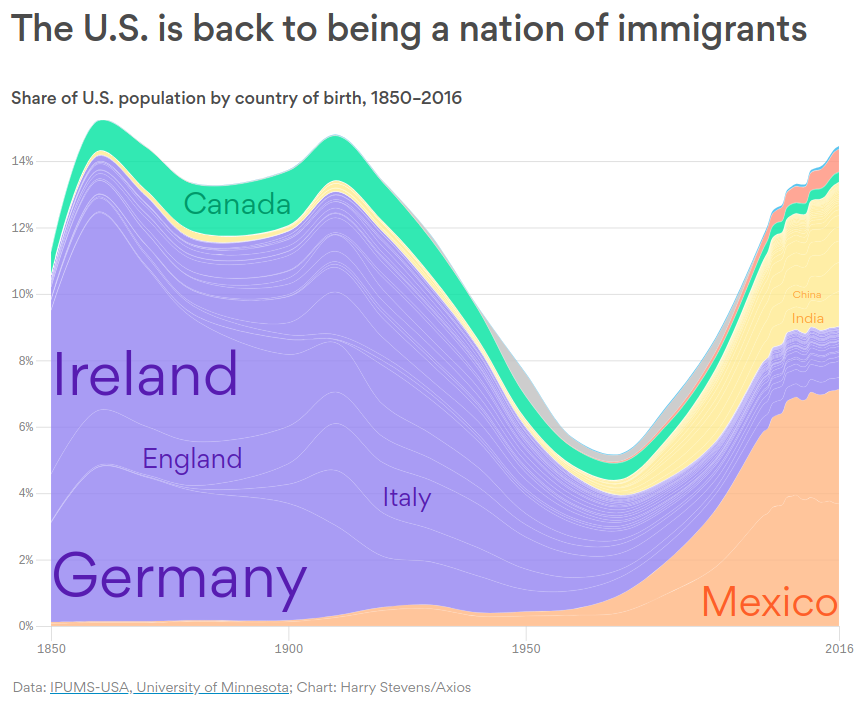{{ message }}

# curran / d3-area-label

A library for placing labels in areas.

Switch branches/tags
Nothing to show

## Files

Failed to load latest commit information.
Type
Name
Commit time

# d3-area-label

A library for placing labels in areas.You can use this to position labels on a StreamGraph or Stacked Area Chart.

Example usage:

```const labels = svg.selectAll('text').data(stacked)
labels
.enter().append('text')
.attr('class', 'area-label')
.merge(labels)
.text(d => d.key)
.attr('transform', d3.areaLabel(area)) // <---------- Call the function like this.```

For more details and context, see test/index.html or run the example on bl.ocks.org.

## How it Works

The label placement algorithm works as follows:

• Measure the width and height of the bounding box of the text.
• Use the bisection method to search for the maximum size rectangle that fits within the area and has the same aspect ratio as the measured bounding box.
• For each iteration of the bisection method (where a specific size is given for testing), loop through all X coordinates that may potentially be used as the left edge of the label bounding box and perform the following test.
• For a given X coordinate to be used as the left edge of the label, `x0`, find the first X coordinate that falls after the right edge of the label `x1`. For each X coordinate `x` between (and including) `x0` and `x1`, compute the `ceiling` and `floor` to be the lowest Y coordinate of the top curve of the area and the highest Y coordinate of the bottom curve of the area, respectively.
• If at any point `(ceiling - floor) < height`, where `height` is the height of the label bounding box being tested, break out of this iteration and move on to testing the next X coordinate.
• If `(ceiling - floor) >= height` after having checked all `x` between `x0` and `x1`, return the current `x` value as the solution. Note Only the first solution found is returned, no attempt is made to optimize this solution, because the optimization occurs at the level of scale choice; the bisection method will converge to a scale for which there is only 1 or very few solutions.
• If no solution was found after having looped through all available X coordinates to be used as the left edge of the label, return `false`.

The set of possible X coordinate to be used as the left edge of the label depends on how interpolate and interpolateResolution are configured. If interpolation is turned off, the original X coordinates from the data points are the only coordinates considered for label placement. For datasets where there are large gaps between X coordinates, we can improve label placement by turning on interpolation, which will generate a certain number (`interpolateResolution`) of evenly spaced X coordinates and use linear interpolation to compute the corresponding Y coordinates for the top and bottom of the area curve. Cranking up the `interpolateResolution` value leads to more optimal label placement at the cost of more computation.

## Installing

If you use NPM, `npm install d3-area-label`. Otherwise, download the latest release.

## API Reference

# d3.areaLabel([area])

Constructs a new label position generator.

If area is specified, invokes areaLabel.area as well (equivalent to `d3.areaLabel().area(area)`).

# areaLabel(data)

Invoke the label position generator with the given data array.

This function computes the optimal position and size for a label and returns an SVG transform string.

# areaLabel.area(area)

Sets the x, y0, and y1 accessors applied to the data array from the given area, an instance of d3.area.

# areaLabel.x(x)

If x is specified, sets the x accessor applied to the data array and returns the label position generator. If x is not specified, returns the current x.

# areaLabel.y0(y0)

If y0 is specified, sets the y0 accessor applied to the data array and returns the label position generator. If y0 is not specified, returns the current y0.

# areaLabel.y1(y1)

If y1 is specified, sets the y1 accessor applied to the data array and returns the label position generator. If y1 is not specified, returns the current y1.

# areaLabel.minHeight(minHeight)

The minimum label bounding box height in pixels. Default is 2.

# areaLabel.epsilon(epsilon)

The tolerance within we wish to optimize the bounding box height (in pixels). Default is 0.01;

# areaLabel.maxIterations(maxIterations)

The maximum number of iterations for the bisection method algorithm, which is used to find the maximum height rectangle that fits within the area.

# areaLabel.interpolate(interpolate)

A boolean value that determines whether or not linear interpolation is used for computing label positions.

If set to false, only X coordinates that correspond to data points are considered for use as the leftmost position of the label bounding box. In cases where there is a high number of evenly spaced data points, a value of false works quite well. When there is a low number of data points, a value of false leads to embarassingly pathetic label placements.

If set to true, then a fixed number of X coordinates (interpolateResolution to be exact), not necessarily corresponding to data points, are considered for use as the leftmost position of the label bounding box. The upper and lower Y values for those X values are imputed from the nearest X values from the data using linear interpolation. When there is a low number of data points, a value of true improves label placement by leaps and bounds. A value of true also leads to more expensive computation for placing labels, so if you're encountering performance problems, try setting this to false.

Default is true.

# areaLabel.interpolateResolution(interpolateResolution)

The integer number of possible X positions to check for placing the leftmost edge of the label bounding box. The X extent of the area is subdivided evenly into this many points. When each point is checked, linear interpolation is used to estimate the data value. Default is 200.

This only comes into effect if interpolate is set to true.

The left padding for labels. This should be a value between 0 and 1. Default is 0.

The right padding for labels. This should be a value between 0 and 1. Default is 0.

The top padding for labels. This should be a value between 0 and 1. Default is 0.

The bottom padding for labels. This should be a value between 0 and 1. Default is 0.

# Thanks

Many thanks to Lee Byron, Noah Veltman, Philippe Rivière, and Adam Pearce for ideas and input.

A library for placing labels in areas.

## Releases 9

All methods chainable Latest
Jul 16, 2019

## Packages 0

No packages published

•
•